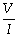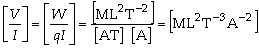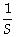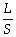# Physics Assignment Help With Resistance

1. Home
2. Physics
3. Electric Current
4. Resistance

## 12.6 Resistance

Resistance is the property of a substance by which it opposes the flow of current through it.

For a given body, the resistance is defined as the ratio of applied potential difference to the resulting current,

R =This property of the substance is due to frequent collisions of electrons with the atoms of the conductor.

SI unit of resistance is named ohm ) which is equivalent to V/A. Its dimensions areW(

[R] =.

### 12.6.1 Dependence of Resistance on Physical Dimensions

For a given body, its resistance is found to be

1. directly proportional to its length (L), and
2. inversely proportional to its area of cross-section (S).

That is, R µ L and R µor R µ= rwhere r is a constant called resistivity.

### 12.6.2 Resistivity or Specific Resistance (r)

The constant r is the characteristic of the material depends on its temperature of which the conductor is made. If L = unity and S = unity, then

R = r

It can also be defined as the resistance of a cube of material of side 1 m when current flows perpendicular to opposite faces.

The SI unit of resistivity is ohm metre (W m).### Email Based Assignment Help in Resistance

We are the leading online Assignment Help provider. Find answers to all of your doubts regarding the Resistance. Assignmenthelp.net provide homework, Assignment Help to the school, college or university level students. Our expert online tutors are available to help you in Resistance. Our service is focused on: time delivery, superior quality, creativity and originality.